NCERT Solutions (Part - 2) - Analysis of Financial Statements

# NCERT Solutions (Part - 2) - Analysis of Financial Statements - Notes | Study Accountancy Class 12 - Commerce

 1 Crore+ students have signed up on EduRev. Have you?

Numerical Questions:
Question 1: Following are the balance sheets of Alpha Ltd. as at March 31st, 2016 and 2017: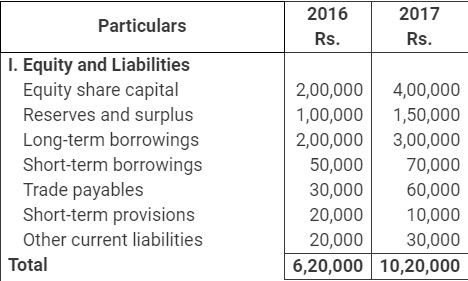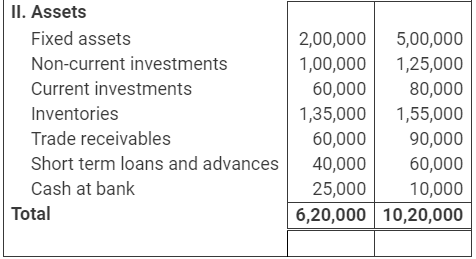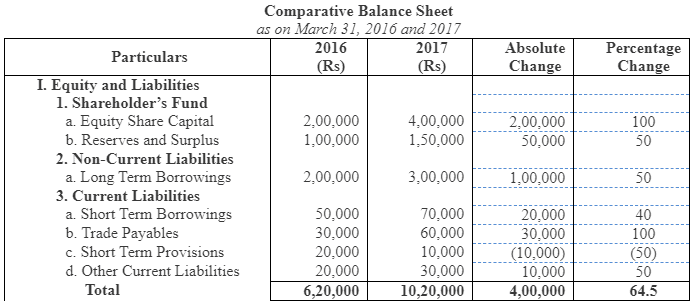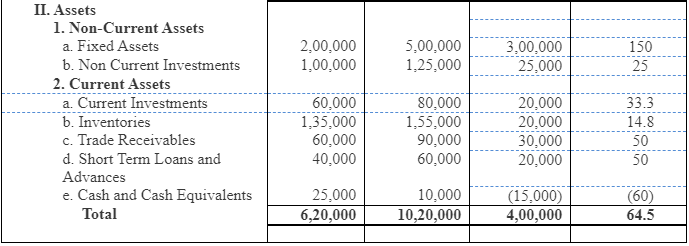Page No 195:
Question 2: Following are the balance sheets of Beta Ltd. at March 31st, 2016 and 2017: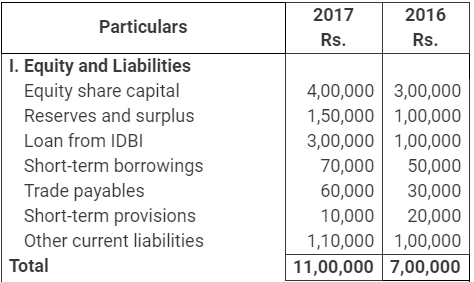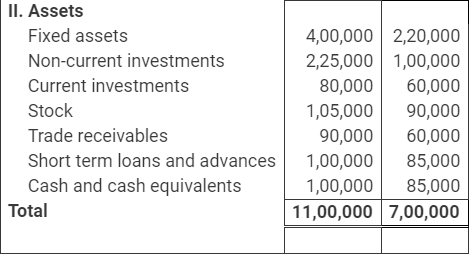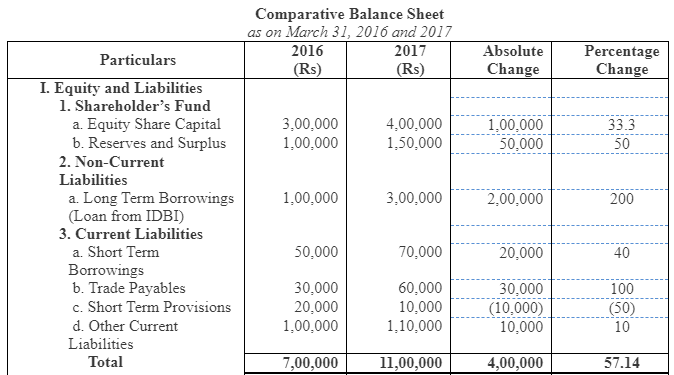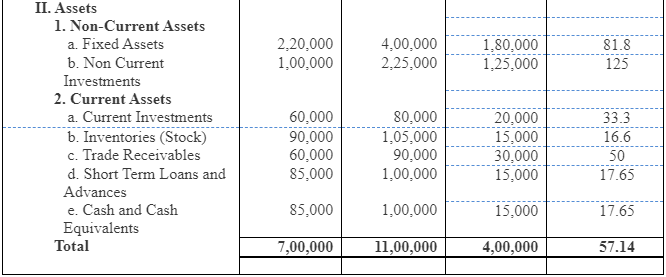Question 3: Prepare Comparative Income Statement from the following information: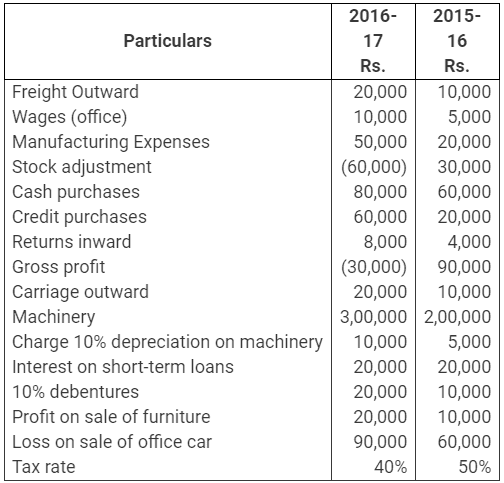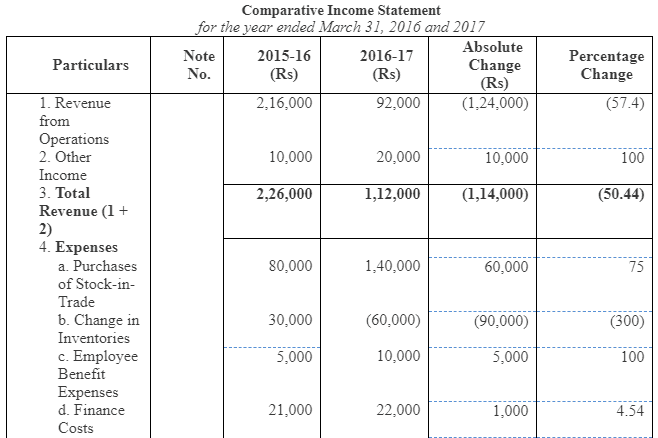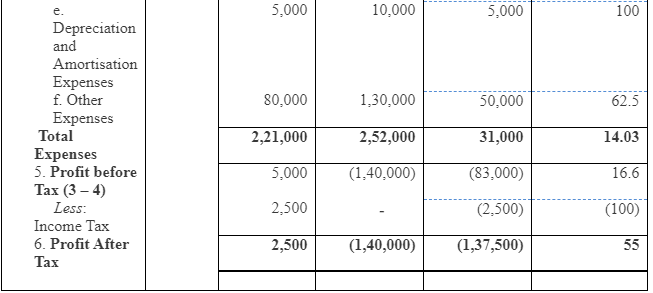Working Notes:
1. Calculation of Net Sales
Net Sales = Cost of Goods Sold + Gross Profit - Sales Return
or, Net Sales = Purchases + Manufacturing Expenses + Change in Inventory + Gross Profit - Sales Return
Net Sales (2016) = 80,000 + 20,000 +30,000 + 90,000 - 4,000 = Rs 2,16,000
Net Sales (2017) = 1,40,000 + 50,000 - 60,000 - 30,000 - 80,000 = Rs 92,000
2. Calculation of Finance Cost
Finance Cost = Interest on short-term loans + Interest on 10% Debentures
Finance Cost (2016) = 20,000 + 1,000 = Rs 21,000
Finance Cost (2017) = 20,000 + 2,000 = Rs 22,000
3. Calculation of Other Expenses
Other Expenses = Freight Outward + Carriage Outward + Loss on sale of office car
Other Expenses (2016) = 10,000 + 10,000 + 60,000 = Rs 80,000
Other Expenses (2017) = 20,000 + 20,000 + 90,000 = Rs 1,30,000

Page No 196:
Question 4:
Prepare Comparative Income Statement from the following information: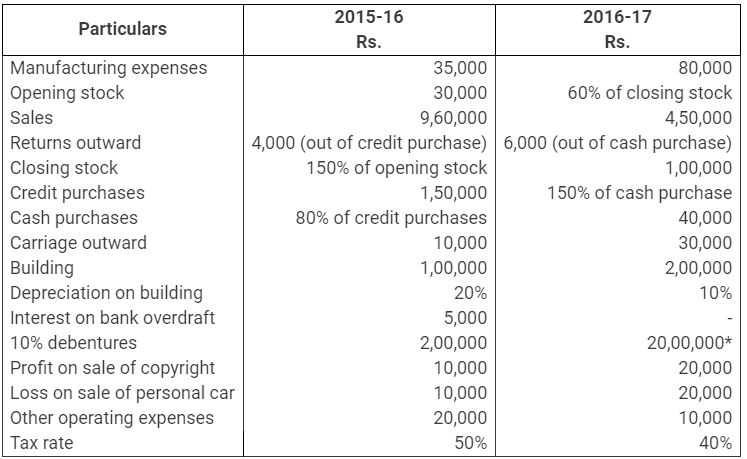*There is a misprint in the book, this should be 2,00,000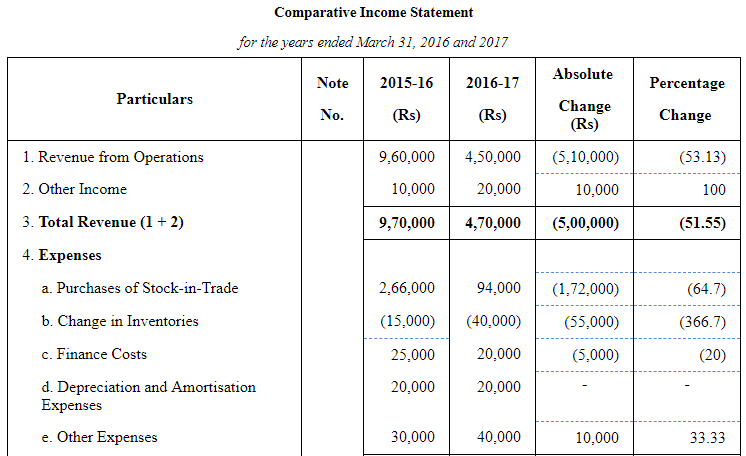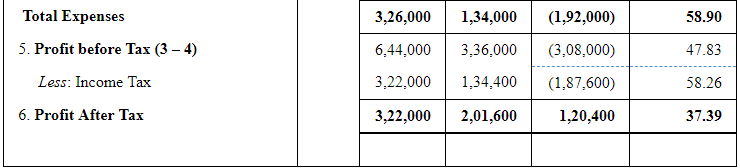Working Notes:
1. Calculation of Net Purchases and Change in Inventory
2. Calculation of Finance Cost
Finance Cost = Interest on Bank Overdraft + Interest on Debentures
Finance Cost (2016) = 5,000 + 20,000 = Rs 25,000
Finance Cost (2017) = 0 + 20,000 = Rs 20,000
3. Calculation of Other Expenses
Other Expenses = Carriage outward + Other operating expenses
Other Expenses (2016) = 10,000 + 20,000 = Rs 30,000
Other Expenses (2017) = 30,000 + 10,000 = Rs 40,000

Page No 197:
Question 5: Prepare a Common-size income statement of Shefali Ltd. with the help of following information: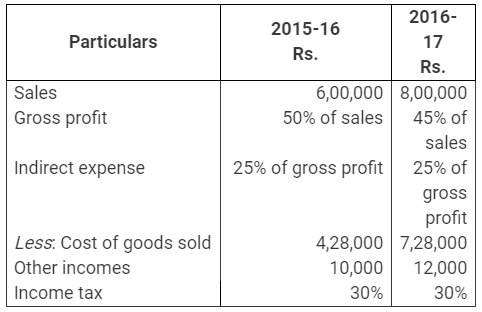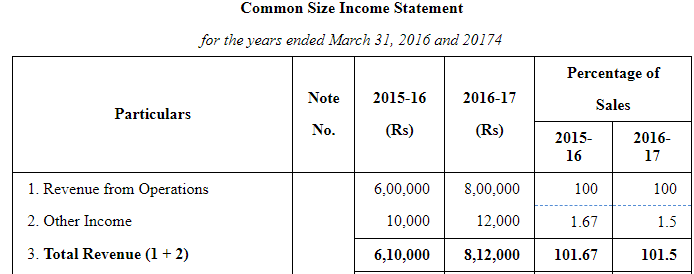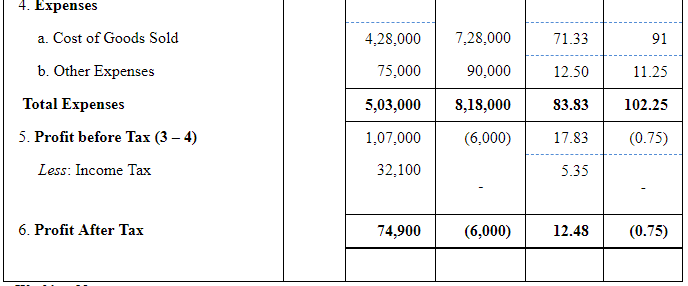Working Notes:
1. Calculation of Other Expenses
Other Expenses = Indirect Expenses = % of Gross Profit
2016=6,00,000×50%×25%=Rs 75,000
2017=8,00,000×45%×25%=Rs 90,000

Question 6: Prepare a Common Size balance sheet from the following balance sheet of Aditya Ltd. and Anjali Ltd.: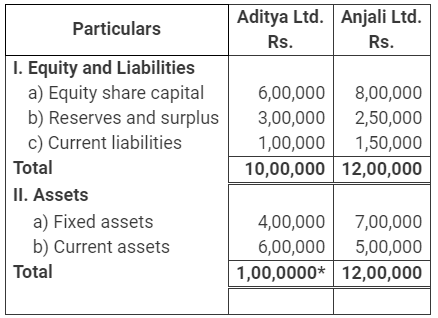*The total of Liabilities side must be equal to the total of Assets side, therefore, it should be 10,00,000.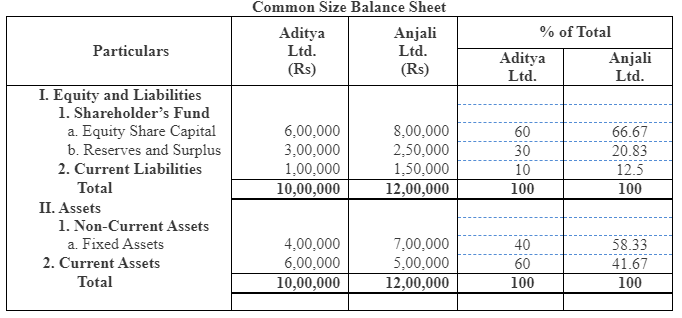The document NCERT Solutions (Part - 2) - Analysis of Financial Statements - Notes | Study Accountancy Class 12 - Commerce is a part of the Commerce Course Accountancy Class 12.
All you need of Commerce at this link: Commerce

## Accountancy Class 12

56 videos|89 docs|68 tests
 Use Code STAYHOME200 and get INR 200 additional OFF

## Accountancy Class 12

56 videos|89 docs|68 tests

Track your progress, build streaks, highlight & save important lessons and more!

,

,

,

,

,

,

,

,

,

,

,

,

,

,

,

,

,

,

,

,

,

;